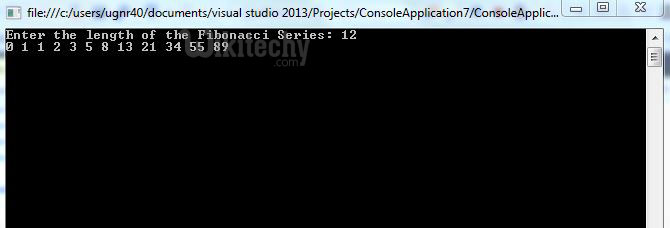# Fibonacci C# - c# - c# tutorial - c# net

## How to write Fibonacci Numbers Program in C# ?

• The Fibonacci Numbers is a sequence of numbers in the following order: 0, 1, 1, 2, 3, 5, 8, 13, 21, 34..
• The next number is found by adding up the two numbers before it. The formula for calculating these numbers is:
• F(n) = F(n-1) + F(n-2)

• where:
• F(n) is the term number.
• F(n-1) is the previous term (n-1).
• F(n-2) is the term before that (n-2).
• By definition, the first two numbers in the Fibonacci Sequence are either 0 and 1, or 1 and 1, depending on the chosen starting point of the sequence and each subsequent number is the sum of the previous two numbers.C# Fibonacci Numbers

## Different ways to print Fibonacci Series in C#?

• In C#, there are several ways to print Fibonacci Series.
• Iterative Approach
• Recursion Approach

## Iterative Approach:

• This is the simplest way of generating Fibonacci series in C#.## Recursive Approach:

• In this approach, we need to pass the length of the Fibonacci Series to the recursive method and then it iterates continuously until it reaches the goal.